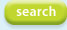# Cacade of containers - simulation model

## Description

Suppose that we have a container with a hole and a time varying inflow. What will happen if we catch the outflow of this container in another container? And this output in another one. Let’s generalize. We have a cascade of N containers where the inflow of container i is the outflow of container i-1 for i =2,.. N. We ignore the delay caused by the distance between the containers, and we assume that the geometry of all containers is the same.

```!
!
t.min = 0;
t.max = 10;
t.step = 0.1;
t.sample = 0.1;
t.method = RK2;
module main
begin
const g   = 9.81, C = 0.62;
real  w_0 = 10,   A = 2.0,
d   = 0.0,  s = 1.25;
const N = 5; ! number of containers;
real f_in(t) = [0, 0, 1, 0, 2, 0, 3, 0, 4, 0, 5, 0, 6, 0, 7, 0, 8, 0, 9, 0, 10, 0];
! For each container:
real w[N](t);    ! volume,
real h[N](t);    ! water level,
real f_out[N](t); ! outflow, and
real v[N](t);     ! velocity at hole.
real w_tot(t);    ! total volume
v(t)     = sqrt(2.0*g*max(h-d, 0));
f_out(t) = C*s*v;
h        = w/max(A,0.001);
w  = integ(f_in - f_out, w_0);
w[i]  = integ(f_out[i-1] - f_out[i], 0), i = 2 to N;
w_tot    = lsum(i = 1 to N, w[i]);
end;
```